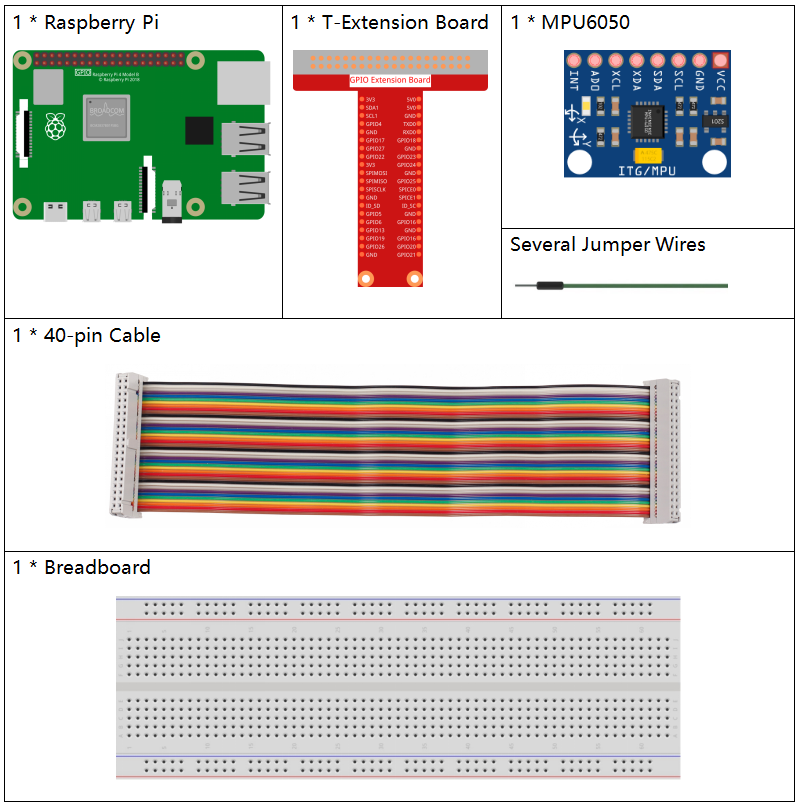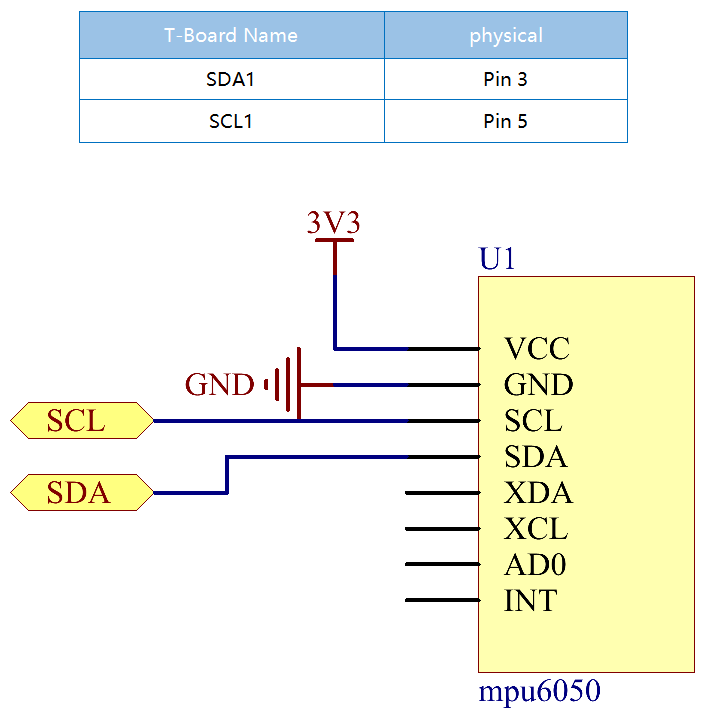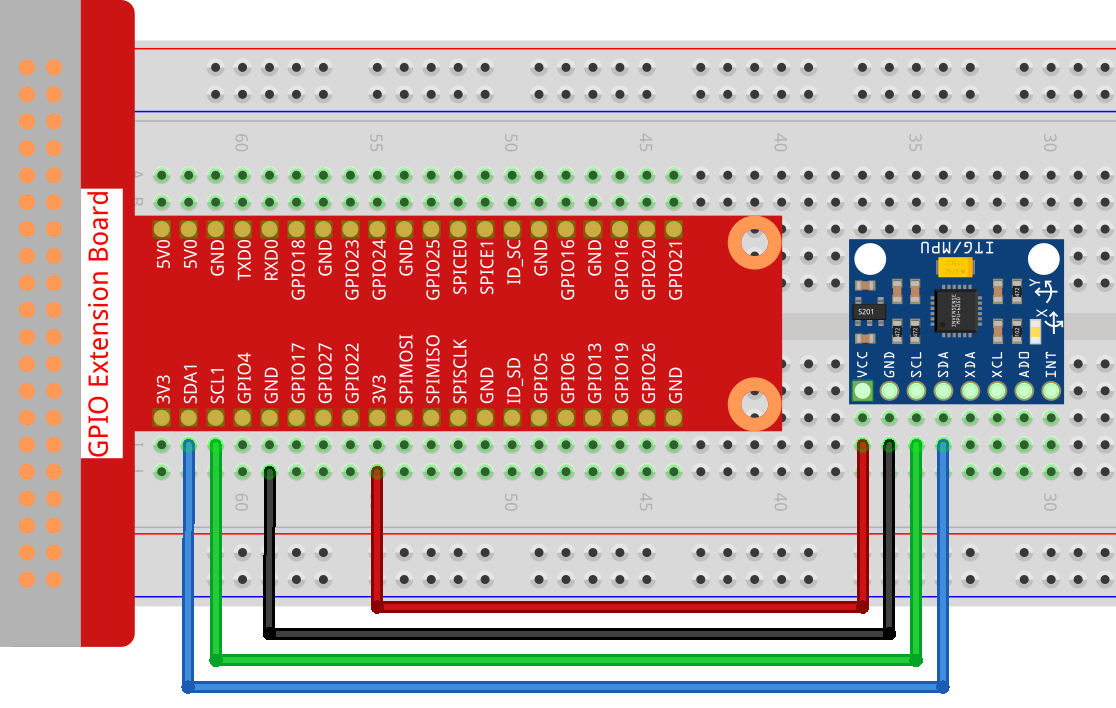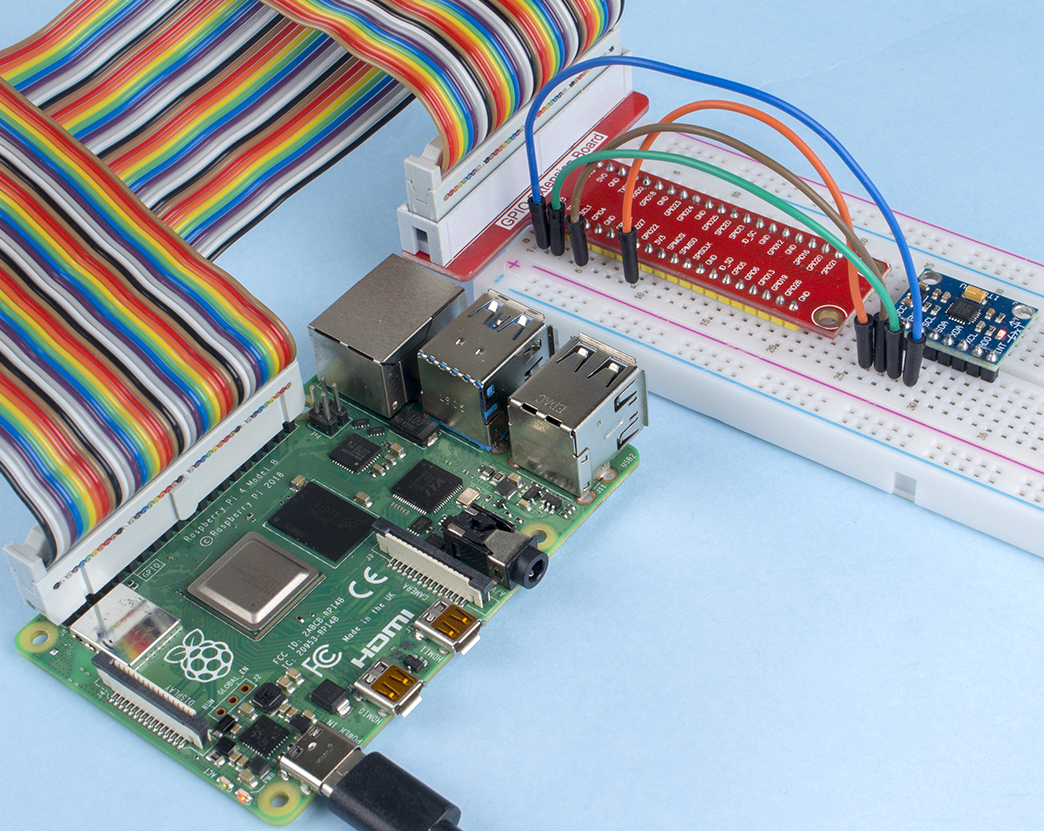# 2.2.9 MPU6050 Module¶

## Introduction¶

The MPU-6050 is the world’s first and only 6-axis motion tracking devices (3-axis Gyroscope and 3-axis Accelerometer) designed for smartphones, tablets and wearable sensors that have these features, including the low power, low cost, and high performance requirements.

In this experiment, use I2C to obtain the values of the three-axis acceleration sensor and three-axis gyroscope for MPU6050 and display them on the screen.

## Required Components¶

In this project, we need the following components.Name

ITEMS IN THIS KIT

Raphael Kit

337

Raphael Kit

COMPONENT INTRODUCTION

GPIO Extension Board

Jumper Wires

MPU6050 Module

## Schematic Diagram¶

MPU6050 communicates with the microcontroller through the I2C bus interface. The SDA1 and SCL1 need to be connected to the corresponding pin.## Experimental Procedures¶

Step 1: Build the circuit.Step 2: Setup I2C (see Appendix I2C Configuration. If you have set I2C, skip this step.)

Step 3: Go to the folder of the code.

```cd ~/raphael-kit/python
```

Step 4: Run the executable file.

```sudo python3 2.2.9_mpu6050.py
```

With the code run, the angle of deflection of the x-axis and y-axis and the acceleration, angular velocity on each axis read by MPU6050 will be printed on the screen after being calculating.

Note

• If you get the error `FileNotFoundError: [Errno 2] No such file or directory: '/dev/i2c-1'`, you need to refer to I2C Configuration to enable the I2C.

• If you get `ModuleNotFoundError: No module named 'smbus2'` error, please run `sudo pip3 install smbus2`.

• If the error `OSError: [Errno 121] Remote I/O error` appears, it means the module is miswired or the module is broken.

Code

Note

You can Modify/Reset/Copy/Run/Stop the code below. But before that, you need to go to source code path like `raphael-kit/python`. After modifying the code, you can run it directly to see the effect.

```import smbus
import math
import time

# Power management registers
power_mgmt_1 = 0x6b
power_mgmt_2 = 0x6c

val = (high << 8) + low
return val

if (val >= 0x8000):
return -((65535 - val) + 1)
else:
return val

def dist(a,b):
return math.sqrt((a*a)+(b*b))

def get_y_rotation(x,y,z):

def get_x_rotation(x,y,z):

bus = smbus.SMBus(1) # or bus = smbus.SMBus(1) for Revision 2 boards

# Now wake the 6050 up as it starts in sleep mode

while True:
time.sleep(0.1)

print ("gyro_xout : ", gyro_xout, " scaled: ", (gyro_xout / 131))
print ("gyro_yout : ", gyro_yout, " scaled: ", (gyro_yout / 131))
print ("gyro_zout : ", gyro_zout, " scaled: ", (gyro_zout / 131))

accel_xout_scaled = accel_xout / 16384.0
accel_yout_scaled = accel_yout / 16384.0
accel_zout_scaled = accel_zout / 16384.0

print ("accel_xout: ", accel_xout, " scaled: ", accel_xout_scaled)
print ("accel_yout: ", accel_yout, " scaled: ", accel_yout_scaled)
print ("accel_zout: ", accel_zout, " scaled: ", accel_zout_scaled)

print ("x rotation: " , get_x_rotation(accel_xout_scaled, accel_yout_scaled, accel_zout_scaled))
print ("y rotation: " , get_y_rotation(accel_xout_scaled, accel_yout_scaled, accel_zout_scaled))

time.sleep(1)
```

Code Explanation

```def read_word(adr):
val = (high << 8) + low
return val

if (val >= 0x8000):
return -((65535 - val) + 1)
else:
return val
```

Read sensor data sent from MPU6050.

```def get_y_rotation(x,y,z):
```

Calculate the deflection angle of the y-axis.

```def get_x_rotation(x,y,z):
```

Calculate the deflection angle of the x-axis.

```gyro_xout = read_word_2c(0x43)

print ("gyro_xout : ", gyro_xout, " scaled: ", (gyro_xout / 131))
print ("gyro_yout : ", gyro_yout, " scaled: ", (gyro_yout / 131))
print ("gyro_zout : ", gyro_zout, " scaled: ", (gyro_zout / 131))
```

Read the values of the x axis, y axis and z axis on the gyroscope sensor, convert the metadata to angular velocity values, and then print them.

```accel_xout = read_word_2c(0x3b)

accel_xout_scaled = accel_xout / 16384.0
accel_yout_scaled = accel_yout / 16384.0
accel_zout_scaled = accel_zout / 16384.0

print ("accel_xout: ", accel_xout, " scaled: ", accel_xout_scaled)
print ("accel_yout: ", accel_yout, " scaled: ", accel_yout_scaled)
print ("accel_zout: ", accel_zout, " scaled: ", accel_zout_scaled)
```

Read the values of the x axis, y axis and z axis on the acceleration sensor, convert the elements to accelerated speed value (gravity unit), and print them.

```print ("x rotation: " , get_x_rotation(accel_xout_scaled, accel_yout_scaled, accel_zout_scaled))
print ("y rotation: " , get_y_rotation(accel_xout_scaled, accel_yout_scaled, accel_zout_scaled))
```

Print the deflection angles of the x-axis and y-axis.

## Phenomenon Picture¶